# Texas Go Math Grade 5 Lesson 7.2 Answer Key Prime and Composite Numbers

Refer to our Texas Go Math Grade 5 Answer Key Pdf to score good marks in the exams. Test yourself by practicing the problems from Texas Go Math Grade 5 Lesson 7.2 Answer Key Prime and Composite Numbers.

## Texas Go Math Grade 5 Lesson 7.2 Answer Key Prime and Composite Numbers

Unlock the Problem

Students are arranging square tables to make one larger, rectangular table at a fundraiser for an animal shelter. If the students want to choose from the greatest number of ways to arrange the tables, should they use 12 or 13 square tables?

• What are the factors of 12?

Use a grid to show all the possible arrangements of 12 and 13 tables.

Label each drawing with the factors modeled.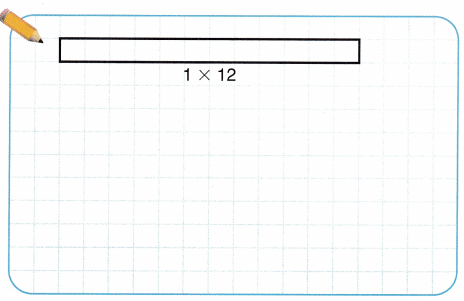The same factors in a different order should be counted only once. For example, 3 × 4 and 4 × 3 are the same factor pair.

So, there are more ways to arrange ___________ tables.

Math Talk
Mathematical Processes

Explain how knowing whether 12 and 13 are prime or helped you solve the problem above.

• A prime number is a whole number greater than 1 that has exactly two factors, 1 and itself.
• A composite number ¡s a whole number greater than 1 that has more than two factors.
Factors of 12: _______ , _______, _______ , _______ , _______ , _____
Factors of 13: _______, _______
12 is a _______ number, and 13 is a _______ number.
Factors of 12: 1 , 2, 3 , 4 , 6, 12
Factors of 13: 1, 13
12 is a composite number, and 13 is a prime number.

Divisibility You can use divisibility rules to help tell whether a number is prime or composite. If a number is divisible by any number other than 1 and itself, then the number is composite.

Math Idea
The number 1 is neither prime nor composite, since it has only one factor: 1.

Tell whether 51 is prime or composite.
Is 51 divisible by 2? _______________________
Is 51 divisible by 3? _______________________
Think: 51 is divisible by a number other than 1 and 51. 51 has more than two factors.
So, 51 is ____________.
Is 51 divisible by 2? No
Is 51 divisible by 3? Yes, 51 ÷ 3 = 17
51 is divisible by a number other than 1 and 51. 51 has more than two factors.
So, 51 is composite number.
Explanation:
51 is not a prime number because there are three factors of 51. By the division method, we find that 1, 3,17, and 51 divide 51 evenly without any remainder. Hence, any number that has more than 2 factors is called as composite number. So, 51 is composite number.

Share and Show

Tell whether the number is prime or composite.

Question 1.
11
Think: Does 11 have other factors besides 1 and itself?
The factors of 11 are 1, 11. So, 11 is a prime number.
Explanation:
11 is a prime number. The number 11 is divisible only by 1 and the number itself. For a number to be classified as a prime number, it should have exactly two factors.

73
The factors of 73 are 1, 73. So, 73 is a prime number.
Explanation:
73 is a prime number. The number 73 is divisible only by 1 and the number itself. For a number to be classified as a prime number, it should have exactly two factors. Since 73 has exactly two factors, 1 and 73, it is a prime number.

Question 3.
69
1 x 69 = 69
3 x 23 = 69
So, 69 is a composite number.
Explanation:
69 is a composite number because 69 has more than 2 factors. The factors of 69 are 1, 3, 23, 69.

Question 4.
42
1 x 42 = 42
2 x 21 = 42
3 x 14 = 42
6 x 7 = 42
So, 42 is a composite number.
Explanation:
42 is a composite number because 42 has more than 2 factors. The factors of 42 are 1, 2, 3, 6, 7, 14, 21, and 42.

Math Talk
Mathematical Processes

Is the product of two prime numbers prime or composite? Explain.

Problem Solving

H.O.T. Analyze Write true or false for each statement. Explain or give an example to support your answer.

Question 5.
The number 1 is not prime.
True, The number 1 is not prime.
Number 1 has positive divisors as 1 and itself. According to the definition of prime numbers, any number having only two positive divisors are known as prime numbers. So,1 is not a prime number and it is not a composite number.

Question 6.
A composite number cannot have three factors.
False, A composite number can have three factors.
Explanation:
Composite numbers are whole numbers that have at least three factors. Prime numbers have two factors one and itself. For example, the only factors for 2 is 1 and 2 (1 x 2). However, 4 has three factors 1, 2, and 4 (1 x 4 and 2 x 2).

Question 7.
Only odd numbers are prime numbers.
False, The prime number 2 is a even number.
Explanation:
A prime number is a positive integer having exactly two factors. If p is a prime, then it’s only factors are necessarily 1 and p itself. The first ten primes are 2, 3, 5, 7, 11, 13, 17, 19, 23, 29.

Question 8.
Every multiple of 7 is a composite number.
False
Multiples of 7: 7, 14, 21, 28, 35, 42, 49, 56, 63, 70…..
Explanation:
The numbers which can be generated by multiplying the two smallest positive integers and containing at least one divisor other than the number ‘1’ and itself are known as composite numbers. These numbers always have more than two factors. The number 7 itself is a multiple of 7 [7 * 1 = 7] and it is not a composite.

H.O.T. Name a 2-digit odd number that is prime. Name a 2-digit odd number that is composite.
The 2-digit odd number 11 is prime number.
The 2-digit odd number 69 is composite number.
Explanation:
The 2 digit means it has two numbers. A prime number is a positive integer having exactly two factors. Prime meaning its factors must be 1 and itself, and it also must be odd number. The 2-digit odd number 11 is prime number.
The 2 digit means it has two numbers. In Mathematics, composite numbers are numbers that have more than two factors. The 2-digit odd number 69 is composite number. 69 is a composite number because 69 has more than 2 factors. The factors of 69 are 1, 3, 23, 69.

Problem Solving

Eratosthenes was a Greek mathematician who lived more than 2,200 years ago. He invented a method of finding prime numbers, which is now called the Sieve of Eratosthenes.

Question 10.
Multi-Step Follow the steps below to circle all prime numbers less than 100. Then list the prime numbers.

STEP 1:
Cross out 1, since 1 is not prime.

STEP 2:
Circle 2, since it is prime. Cross out all other multiples of 2.

STEP 3:
Circle the next number that is not crossed out. This number is prime. Cross out all the multiples of this number.

STEP 4:
Repeat Step 3 until every number is either circled or crossed out.So, the prime numbers less than 100 are _______________So, the prime numbers less than 100 are 2, 3, 5, 7, 11, 13, 17, 19, 23, 29, 31, 37, 41, 43, 47, 53, 59, 61, 67, 71, 73, 79, 83, 89, 97.

Question 11.
H.O.T. Explain why the multiples of any number other than 1 are not prime numbers.Question 12.
Reasoning Talia’s locker combination consists of three prime numbers. The sum of these numbers is also a prime number. Which of these might be her combination?
(A) 3 – – 8 – – 17
(B) 2 – – 3- -19
(C) 7 – – 13 – – 3
(D) 11 – – 2 – – 5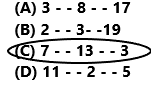7 + 13 + 3 = 23
The sum of above prime numbers is also a prime number.
So, option C is correct.
Explanation:
Talia’s locker combination consists of three prime numbers. The sum of these numbers is also a prime number. The combination is 7 – – 13 – – 3. Add these three prime numbers the sum is 23. 23 is also a prime number. So, option C is correct.

Question 13.
A certain number is a whole number. If the number is also composite, what must be true about the number?
(A) It is odd.
(B) It has more than two factors.
(C) It has exactly two factors.
(D) It has two or more digits.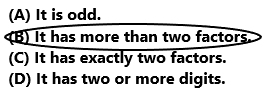So, option B is correct.
Explanation:
A certain number is a whole number. If the number is also composite then it has more than two factors. So, option B is correct.

5th Grade Go Math Practice and Homework Lesson 7.2 Answer Key Question 14.
Multi-Step The sum of three prime numbers is 12. What could the numbers be?
(A) 3, 7, 2
(B) 6, 6, 0
(C) 3, 5, 7
(D) 3, 4, 5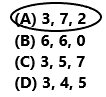3 + 7 + 2 = 12
So, option A is correct.
Explanation:
The three prime numbers are 3, 7, 2. The sum of these three prime numbers is 12. So, option A is correct.

Texas Test Prep

Question 15.
The number 2 is
(A) prime.
(B) composite.
(C) neither prime nor composite.
(D) both prime and composite.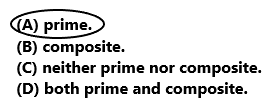So, option A is correct.
Explanation:
The number 2 is a prime number. So, option A is correct.

### Texas Go Math Grade 5 Lesson 7.2 Homework and Practice Answer Key

Tell whether the number is prime or composite.

Question 1.
19
1 x 19 = 19
So, the number 19 is a prime number.
Explanation:
Prime numbers are the positive integers having only two factors, 1 and the integer itself. The factors of 19 are 1, 19. So the number 19 is a prime number.

Question 2.
81
The factors of 81 are 1, 3, 9, 27, 81
So, the number 81 is a composite number.
Explanation:
A composite number is a number that can be divided evenly by more numbers than 1 and itself. It is the opposite of a prime number. The number 81 can be evenly divided by 1, 3, 9, 27 and 81, with no remainder. Since 81 cannot be divided by just 1 and 81. So, it is a composite number.

Question 3.
52
The factors of 52 are 1, 2, 4, 13, 26, 52.
So, the number 52 is a composite number.
Explanation:
The number 52 has more than two factors. The factors of 52 are 1, 2, 4, 13, 26, 52. In other words, 52 is a composite number because 52 has more than 2 factors.

Question 4.
23
1 x 23 = 23
So, the number 23 is a prime number.
Explanation:
Prime numbers are the positive integers having only two factors, 1 and the integer itself. The factors of 23 are 1, 23. So the number 23 is a prime number.

Question 5.
33
The factors of 33 are 1, 3, 11, 33.
So, the number 33 is a composite number.
Explanation:
A composite number is a number that can be divided evenly by more numbers than 1 and itself. The number 33 can be evenly divided by 1, 3, 11 and 33, with no remainder. Since 33 cannot be divided by just 1 and 33. So, 33 is a composite number.

60
The factors of 60 are 1, 2, 3, 4, 5, 6, 10, 12, 15, 20, 30, 60.
So, the number 60 is a composite number.
Explanation:
A composite number is a number that can be divided evenly by more numbers than 1 and itself. The number 60 can be evenly divided by 1, 2, 3, 4, 5, 6, 10, 12, 15, 20, 30, 60, with no remainder. Since 60 cannot be divided by just 1 and 60. So, 60 is a composite number.

Question 7.
31
1 x 31 = 31
So, the number 31 is a prime number.
Explanation:
Prime numbers are the positive integers having only two factors, 1 and the integer itself. The factors of 31 are 1, 31. So the number 31 is a prime number.

Question 8.
25
The factors of 25 are 1, 5, 25.
So, the number 25 is a composite number.
Explanation:
A composite number is a number that can be divided evenly by more numbers than 1 and itself. The number 25 can be evenly divided by 1, 5, 25, with no remainder. Since 25 cannot be divided by just 1 and 25. So, 25 is a composite number.

Write true or false for each statement. Explain or give an example to support your answer.

Question 9.
A prime number is always greater than 1.
True, A prime number is always greater than 1.
Prime numbers are 2, 3, 5, 7, 11, 13…..
Explanation:
A prime number is a whole number greater than 1. It has exactly two factors, that is, 1 and the number itself. There is only one even prime number, that is, 2. Any two prime numbers are always co-prime to each other. So the given statement is True.

Question 10.
The number 17 is a prime number.
True, The number 17 is a prime number.
Explanation:
A prime number is a whole number greater than 1. It has exactly two factors, that is, 1 and the number itself. The factors of 17 is 1, 17. The number 17 is a prime number. So the given statement is True.

Question 11.
Every multiple of 5 is a composite number.
True, Every multiple of 5 is a composite number.
Explanation:
In Mathematics, composite numbers are numbers that have more than two factors. The multiples of 5 are 10, 15, 20, 25, 30, 35 and so on. The above-given statement is true. Every multiple of 5 is a composite number. Except for 5 because 5 is a prime number.

Practice and Homework Lesson 7.2 Go Math Grade 5 Question 12.
A number can be both prime and composite.
False, A number cannot be both prime and composite numbers.
Explanation:
Numbers can be classified on the basis of the number of factors they have. If a number has just two factors – 1 and the number itself, then it is a prime number. However, most numbers have more than two factors, and they are called composite numbers. The opposite of composite is prime numbers. So, the above-given statement is false.

Problem Solving

Question 13.
The students in math class use square tiles to make arrays. Celia says they can make more arrays with 8 tiles than with 9 tiles because 8 has more factors. Is Celia correct? Explain.

Lesson Check

Question 14.
Four boys compare the numbers on their football jerseys. Parker has the number 55. Nick has 47. Marshall has 16, and Leon has 9. Whose jersey has a prime number?
(A) Parker
(B) Nick
(C) Marshall
(D) Leon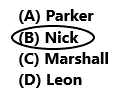The number on the Nick jersey is 47. Where 47 is a prime number.
So, option B is correct.
Explanation:
Four boys compare the numbers on their football jerseys. Parker has the number 55. Nick has 47. Marshall has 16, and Leon has 9. The number on the Nick jersey is 47. The factors of 47 is 1, 47. Where 47 is a prime number. So, option B is correct.

Question 15.
Harlan played her favorite game app three times this morning. In each game, the number of points she scored was a prime number. When she adds the points for the three games together, the sum is also a prime number. Which of these might be her scores?
(A) 17, 19, 20
(B) 11, 17, 21
(C) 21, 23, 13
(D) 29, 19, 23She scored 29, 19, 23 points in each game.
29 + 19 + 23 = 71
The points 29, 19, 23 are prime numbers. The sum of these three points is 71.  It is also a prime number.
So, option D is correct.
Explanation:
Harlan played her favorite game app three times this morning. In each game, the number of points she scored was a prime number. She scored 29, 19, 23 points in each game. When she adds the points for the three games together, the sum is also a prime number. The sum of these three points is 71. So, option D is correct.

Question 16.
Esteban made a list of prime numbers less than 40. He listed the numbers in order from least to greatest. Which number did Esteban put on his list after 29?
(A) 41
(B) 23
(C) 33
(D) 31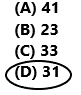The prime number that Esteban put on his list after 29 is 31.
So, option D is correct.
Explanation:
Esteban made a list of prime numbers less than 40. He listed the numbers in order from least to greatest. The prime numbers less than 40 are 2, 3, 5, 7, 11, 13, 17, 19, 23, 29, 31, 37. The prime number that Esteban put on his list after 29 is 31. So, option D is correct.

Question 17.
Carolyn found that the difference between two prime numbers is a composite number. Which could be the prime numbers?
(A) 2, 5
(B) 13, 2
(C) 23, 19
(D) 13, 11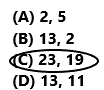23 – 19 = 4
The number 4 is a composite number.
So, option C is correct.
Explanation:
Carolyn found that the difference between two prime numbers is a composite number. The prime numbers are 23, 19. Subtract 19 from 23 the difference is 4. The number 4 is a composite number. So, option C is correct.

Multi-Step Trisha spins a spinner. The pointer lands on three prime numbers. She says the sum is less than 12. Which could be the numbers?
(A) 2, 3, 5
(B) 2, 4, 5
(C) 2, 7, 3
(D) 0, 9, 1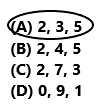The three prime numbers are 2, 3, 5.
2 + 3 + 5 = 10
So, option A is correct.
Explanation:
Trisha spins a spinner. The pointer lands on three prime numbers. The three prime numbers are 2, 3, 5. She says the sum is less than 12. Add 2 with 3 and 5 the sum is 10. So, option A is correct.

Question 19.
Multi-Step Felix found the sum of two prime numbers and one composite number to be 45. The difference between the greatest and least number is 4. Which could be the three numbers?
(A) 13, 17, 15
(B) 18, 14, 13
(C) 15, 14, 16
(D) 11, 19, 12